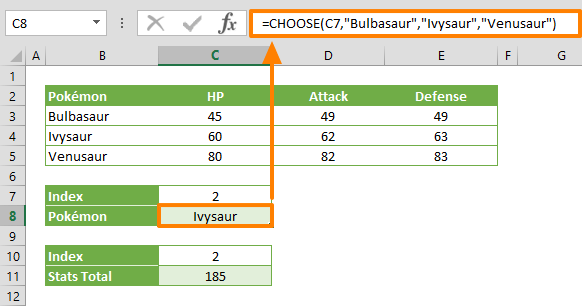The Excel CHOOSE function is a Lookup & Reference formula that can return the value of one of its arguments by a given position. For example, if the value arguments are the months of a year, the CHOOSE function can return one of the months, when a number between 1 and 12 is used for the index_num argument. In this guide, we’re going to show you how to use the Excel CHOOSE function and also go over some tips and error handling methods.

## Supported versions

• All Excel versions

## Excel CHOOSE Function Syntax

CHOOSE(index_num, value1, [value2], …)

## Arguments

 index_num A number indicates which valueN argument will be returned. If index_num is 1, the function returns value1; if it is 3, the function returns value3; and so on. If index_num has decimal digits, it is truncated. value1, [value2], … Values to return. Only value1 is required. You can set up to 254 values.

## Excel CHOOSE Function Examples

### Basic Example

The following formula has 3 value arguments, in addition to a index_num argument. If we set the index_num argument to 2, the second value argument, value2, will be returned by the function.

=CHOOSE(2,"Bulbasaur","Ivysaur","Venusaur") returns "Ivysaur"### Return Reference

You can set cell or range references to the value arguments. If you assign range references to the value arguments, the CHOOSE function returns the entire range, based on the given index_num value. You can use this range reference with any function that accepts an array, like the SUM or VLOOKUP functions.## Excel CHOOSE Function Tips

• The Excel CHOOSE function can get up to 254 value arguments. In this case, prefer using a lookup function to deal with high number of inputs instead.
• The index_num argument can be an array of numbers as well. If so, every value is evaluated when the CHOOSE function is executed.

## Issues

• If index_num is less than 1 or greater than the number of the last value in the list, CHOOSE function returns the #VALUE! error value.